• ## MATLAB三维绘图

千次阅读 2020-07-19 15:14:50
MATLAB绘图篇—三维绘图 目录MATLAB绘图篇—三维绘图1.三维曲线2.三维曲面三维曲面绘制过程（1）生成平面网格坐标矩阵（2）利用绘制三维曲面函数绘制曲面3.其他三维曲面 1.三维曲线 绘制三维曲线的基本函数 调用格式...
MATLAB三维绘图

目录
MATLAB三维绘图1.三维曲线2.三维曲面三维曲面绘制过程（1）生成平面网格坐标矩阵（2）利用绘制三维曲面函数绘制曲面

3.其他三维曲面

1.三维曲线
绘制三维曲线的基本函数
调用格式为： plot3(x1,y1,z1,选项1,x2,y2,z2,选项2,…,xn,yn,zn,选项n) 其中每一组x,y,z组成一组曲线的坐标参数，选项的定义和plot函数相同。
（1）当x,y,z是同维向量时，则x,y,z 对应元素构成一条三维曲线。 （2）当x,y,z是同维矩阵时，则以x,y,z对应列元素绘制三维曲线，曲线条数等于矩阵列数。
例：绘制空间曲线
t=0:pi/50:2*pi;
x=8*cos(t);
y=4*sqrt(2)*sin(t);
z=-4*sqrt(2)*sin(t);
plot3(x,y,z);

输出结果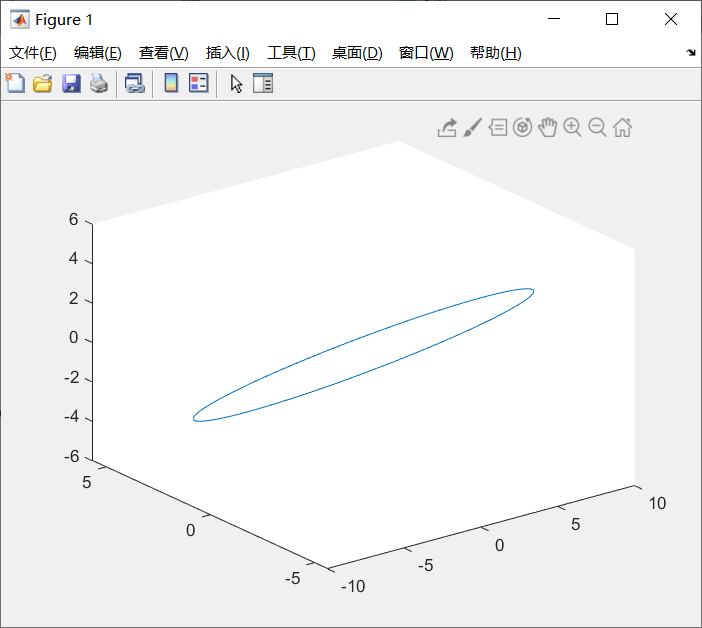2.三维曲面
三维曲面绘制过程
（1）生成平面网格坐标矩阵
过程： ① 在xy平面选定一矩形区域 D=[a,b]×[c,d]； ② 将[a,b]在x方向分成m份，将[c,d]在y方向分成n份，由各划分点分别作平行于两坐标轴的直线，将区域D分成m×n个小矩形，生成代表每一个小矩形顶点坐标的平面网格坐标矩阵；
生成方法： ①利用矩阵运算生成
x=a:dx:b; y=(c:dy:d)';
X=ones(size(y))*x;
Y=y*ones(size(x));

②利用meshgrid函数生成
x=a:dx:b; y=c:dy:d;
[X,Y]=meshgrid(x,y);

（2）利用绘制三维曲面函数绘制曲面
绘制函数：
①mesh函数 用于绘制三维网格图。在不需要绘制特别精细的三维曲面图时，可以通过网格图来表示三维曲面。 调用格式：mesh(x,y,z,c)
②surf函数 用于绘制三维曲面图，各线条之间的补面用颜色填充。 调用格式：surf(x,y,z,c)
③plot3函数
注：一般情况下，x,y,z是维数相同的矩阵，x,y是网格坐标矩阵，z是网格点上的高度矩阵。c称为色标矩阵，用于指定在不同高度下的颜色范围。当c省略时，c=z,颜色的设定是正比于图形的高度（z值）的，这样就可以得出层次分明的三维图形。 当x,y省略时，把z矩阵的列下标当作x轴坐标，行下标作为y轴坐标，然后绘制三维曲面图。 当x，y是向量时，要求x的长度等于z矩阵的列数，y的长度等于z矩阵的行数，x、y向量元素的组合构成网格点的x、y坐标，z坐标则取自z矩阵，然后绘制三维曲面图。
例:绘制z=sin(y)cos(x)所表示的曲面
x=0:0.1:2*pi;
[x,y]=meshgrid(x);			%生成平面网格坐标矩阵
z=sin(y).*cos(x);
mesh(x,y,z);					%绘制曲面
xlabel('x');				%标明x轴名字
ylabel('y');
zlabel('z');
title('mesh');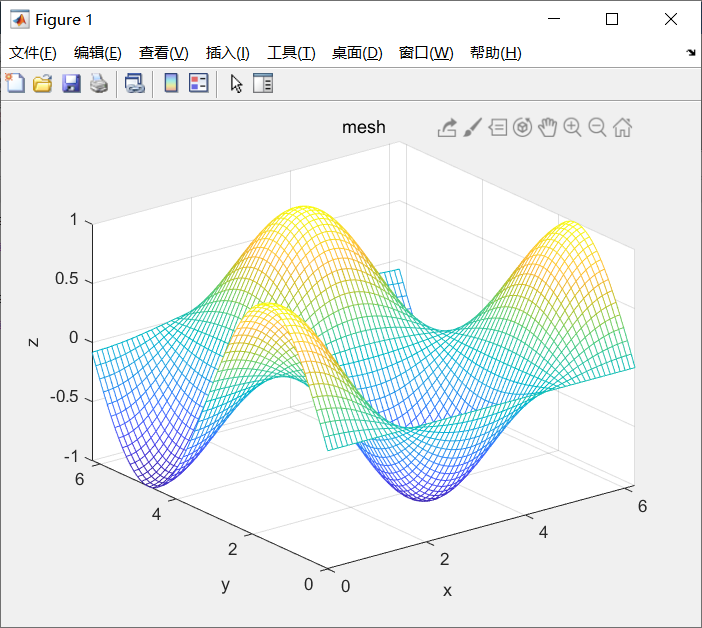x=0:0.1:2*pi;
[x,y]=meshgrid(x);
z=sin(y).*cos(x);
surf(x,y,z);
xlabel('x')；
ylabel('y')；
zlabel('z');
title('surf');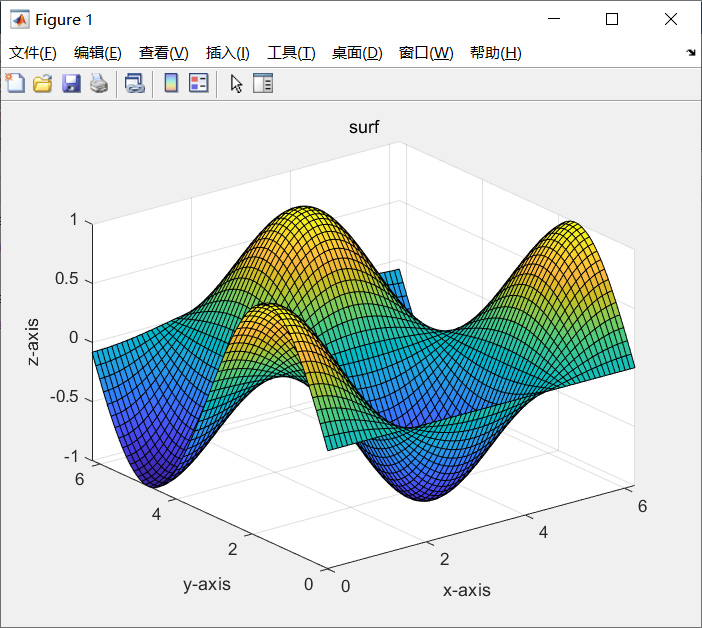x=0:0.1:2*pi;
[x,y]=meshgrid(x);
z=sin(y).*cos(x);
plot3(x,y,z);
xlabel('x');
ylabel('y');
zlabel('z');
title('plot3-1');
grid;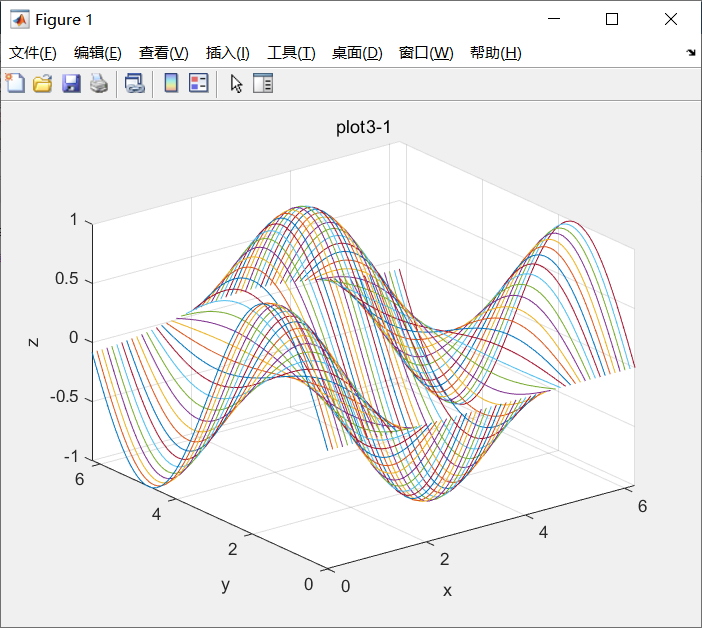3.其他三维曲面
表
函数图形bar3(y) ; bar3(x,y)三维条形图pie3(x,explode)三维饼图fill3(x,y,z,c)三维实心图scatter3(x,y,z,c)三维散点图stem3(z);stem3(x,y,z)三维杆图quiver3(x,y,z,u,v,w)三维箭头图
展开全文• ## matlab矩阵绘三维图

万次阅读 2018-09-26 10:37:22
2.画矩阵三维图     clc; clear all; close all; X=[0 1 2 3 4 5 6 7 8 9]; Y=[0 1 2 3 4 5 6 7 8 9]; for i=1:1:length(X) for j=1:1:...
axis off;% 去掉坐标轴
axistight;% 紧坐标轴
axisequal;% 等比坐标轴
axis([-0.1, 8.1, -1.1, 1.1]);% 坐标轴的显示范围

% gca: gca, h=figure(...);
set(gca,'XLim',[3 40]);% X轴的数据显示范围
set(gca,'XTick',[-3.14,0,3.14] );% X轴的记号点
set(gca,'XTicklabel',{'-pi','0','pi'});% X轴的记号
set(gca,'XTick', []);% 清除X轴的记号点
set(gca,'XGrid','on');% X轴的网格
set(gca,'XDir','reverse');% 逆转X轴
set(gca,'XColor','red');% X轴的颜色
1. axis([xmin xmax ymin ymax])
设置当前图形的坐标范围，分别为x轴的最小、最大值，y轴的最小最大值
2. V=axis
返回包含当前坐标范围的一个行向量
3. axis auto
将坐标轴刻度恢复为自动的默认设置
4. axis manual
冻结坐标轴刻度，此时如果hold被设定为on，那么后边的图形将使用与前面相同的坐标轴刻度范围
5. axis tight
将坐标范围设定为被绘制的数据范围
6. axis fill
这是坐标范围和屏幕的高宽比，使得坐标轴可以包含整个绘制的区域。该选项只有在PlotBoxaApectRatio或DataAspectRatioMode被设置为‘manual’模式才有效
7. axis ij
将坐标轴设置为矩阵模式。此时水平坐标轴从左到有取值，垂直坐标从上到下
8. axis xy
将坐标设置为笛卡尔模式。此时水平坐标从左到右取值，垂直坐标从下到上取值
9. axis equal
设置屏幕高宽比，使得每个坐标轴的具有均匀的刻度间隔
10. axis square
将坐标轴设置为正方形
11. axis normal
将当前的坐标轴框恢复为全尺寸，并将单位刻度的所有限制取消
12. axis vis3d
冻结屏幕高宽比，使得一个三维对象的旋转不会改变坐标轴的刻度显示
13. axis off
关闭所有的坐标轴标签、刻度、背景
14. axis on
打开所有的坐标轴标签、刻度、背景
--------------------- 本文来自 还在学习的编程小白 的CSDN 博客 ，全文地址请点击：https://blog.csdn.net/qq_35052417/article/details/76461643?utm_source=copy

1.画函数的三维图，如：Z(X,Y)=2*X.*exp(-X.^2-Y.^2)+1;

close all;  [X,Y]=meshgrid(-2:0.5:2,-2:0.5:2);%生成坐标轴  Z=2*X.*exp(-X.^2-Y.^2)+1;%Z是X,Y的函数  %%%%%%%%%%%%%%%%%%%%%%%%%%%%%%%%%%%%%%%%%%%%%%%%%%%%%%%%%%%%%%%  num=0;  num=num+1;  subplot(2,3,num);  plot3(X,Y,Z);  axis([-3 3 -3 3 0 2]);%限定显示的范围  xlabel('x轴');%x轴坐标  ylabel('y轴');%y轴坐标  zlabel('z轴');%z轴坐标  title('http://blog.csdn.net/nuptboyzhb/ figure(1)');%标题  %%%%%%%%%%%%%%%%%%%%%%%%%%%%%%%%%%%%%%%%%%%%%%%%%%%%%%%%%%%%%%%  num=num+1;  subplot(2,3,num);  mesh(X,Y,Z);  axis([-3 3 -3 3 0 2]);%限定显示的范围  xlabel('x轴');%x轴坐标  ylabel('y轴');%y轴坐标  zlabel('z轴');%z轴坐标  title('http://blog.csdn.net/nuptboyzhb/ figure(2)');%标题  %%%%%%%%%%%%%%%%%%%%%%%%%%%%%%%%%%%%%%%%%%%%%%%%%%%%%%%%%%%%%%%  num=num+1;  subplot(2,3,num);  meshc(X,Y,Z);  axis([-3 3 -3 3 0 2]);%限定显示的范围  xlabel('x轴');%x轴坐标  ylabel('y轴');%y轴坐标  zlabel('z轴');%z轴坐标  title('http://blog.csdn.net/nuptboyzhb/ figure(3)');%标题  %%%%%%%%%%%%%%%%%%%%%%%%%%%%%%%%%%%%%%%%%%%%%%%%%%%%%%%%%%%%%%%  num=num+1;  subplot(2,3,num);  surf(X,Y,Z);  axis([-3 3 -3 3 0 2]);%限定显示的范围  xlabel('x轴');%x轴坐标  ylabel('y轴');%y轴坐标  zlabel('z轴');%z轴坐标  title('http://blog.csdn.net/nuptboyzhb/ figure(4)');%标题  %%%%%%%%%%%%%%%%%%%%%%%%%%%%%%%%%%%%%%%%%%%%%%%%%%%%%%%%%%%%%%%  num=num+1;  subplot(2,3,num);  meshz(X,Y,Z);  axis([-3 3 -3 3 0 2]);%限定显示的范围  xlabel('x轴');%x轴坐标  ylabel('y轴');%y轴坐标  zlabel('z轴');%z轴坐标  title('http://blog.csdn.net/nuptboyzhb/ figure(5)');%标题  %%%%%%%%%%%%%%%%%%%%%%%%%%%%%%%%%%%%%%%%%%%%%%%%%%%%%%%%%%%%%%%  num=num+1;  subplot(2,3,num);  surf(X,Y,Z);  hold on;  stem3(X,Y,Z,'r');%画竖线  axis([-3 3 -3 3 0 2]);%限定显示的范围  xlabel('x轴');%x轴坐标  ylabel('y轴');%y轴坐标  zlabel('z轴');%z轴坐标  title('http://blog.csdn.net/nuptboyzhb/ figure(6)');%标题2.画矩阵的三维图

clc;  clear all;  close all;  X=[0 1 2 3 4 5 6 7 8 9];  Y=[0 1 2 3 4 5 6 7 8 9];  for i=1:1:length(X)  for j=1:1:length(Y)  Z(i,j)=mod(i*j*rand(1),9);  end  end  %%%%%%%%%%%%%%%%%%%%%%%%%%%%%%%%%%%%%%%%%%%%%%%%%%%%%%%%%%%%%%%  num=0;  num=num+1;  subplot(2,3,num);  plot3(X,Y,Z);  axis([0 9 0 9 0 9]);%限定显示的范围  xlabel('x轴');%x轴坐标  ylabel('y轴');%y轴坐标  zlabel('z轴');%z轴坐标  title('http://blog.csdn.net/nuptboyzhb/ figure(1)');%标题  %%%%%%%%%%%%%%%%%%%%%%%%%%%%%%%%%%%%%%%%%%%%%%%%%%%%%%%%%%%%%%%  num=num+1;  subplot(2,3,num);  mesh(X,Y,Z);  axis([0 9 0 9 0 9]);%限定显示的范围  xlabel('x轴');%x轴坐标  ylabel('y轴');%y轴坐标  zlabel('z轴');%z轴坐标  title('http://blog.csdn.net/nuptboyzhb/ figure(2)');%标题  %%%%%%%%%%%%%%%%%%%%%%%%%%%%%%%%%%%%%%%%%%%%%%%%%%%%%%%%%%%%%%%  num=num+1;  subplot(2,3,num);  meshc(X,Y,Z);  axis([0 9 0 9 0 9]);%限定显示的范围  xlabel('x轴');%x轴坐标  ylabel('y轴');%y轴坐标  zlabel('z轴');%z轴坐标  title('http://blog.csdn.net/nuptboyzhb/ figure(3)');%标题  %%%%%%%%%%%%%%%%%%%%%%%%%%%%%%%%%%%%%%%%%%%%%%%%%%%%%%%%%%%%%%%  num=num+1;  subplot(2,3,num);  surf(X,Y,Z);  axis([0 9 0 9 0 9]);%限定显示的范围  xlabel('x轴');%x轴坐标  ylabel('y轴');%y轴坐标  zlabel('z轴');%z轴坐标  title('http://blog.csdn.net/nuptboyzhb/ figure(4)');%标题  %%%%%%%%%%%%%%%%%%%%%%%%%%%%%%%%%%%%%%%%%%%%%%%%%%%%%%%%%%%%%%%  num=num+1;  subplot(2,3,num);  meshz(X,Y,Z);  axis([0 9 0 9 0 9]);%限定显示的范围  xlabel('x轴');%x轴坐标  ylabel('y轴');%y轴坐标  zlabel('z轴');%z轴坐标  title('http://blog.csdn.net/nuptboyzhb/ figure(5)');%标题  %%%%%%%%%%%%%%%%%%%%%%%%%%%%%%%%%%%%%%%%%%%%%%%%%%%%%%%%%%%%%%%  num=num+1;  subplot(2,3,num);  surf(X,Y,Z);  hold on;  stem3(X,Y,Z,'r');%画竖线  axis([0 9 0 9 0 9]);%限定显示的范围  xlabel('x轴');%x轴坐标  ylabel('y轴');%y轴坐标  zlabel('z轴');%z轴坐标  title('http://blog.csdn.net/nuptboyzhb/ figure(6)');%标题3.画矩阵的颜色深度图
我自己编写的一个函数：graycolor.m
%filename：graycolor.m %郑海波 2013-01-31 %http://blog.csdn.net/nuptboyzhb/ %原理：灰度图像的伪彩色处理 %值越大，显示的颜色越蓝，越小，显示的颜色越红！ %参数：newbuf必须使二维数组或矩阵 function graycolor(newbuf); min_n=min(min(newbuf)); max_n=max(max(newbuf)); newbuf=newbuf./(max_n-min_n)*255; [M N]=size(newbuf); for i=1:1:M     for j=1:1:N         R(i,j)=GrayColorR(newbuf(i,j));         G(i,j)=GrayColorG(newbuf(i,j));         B(i,j)=GrayColorB(newbuf(i,j));     end end img(1:1:M,1:1:N,1)=R(1:M,1:N); img(1:1:M,1:1:N,2)=G(1:M,1:N); img(1:1:M,1:1:N,3)=B(1:M,1:N); imshow(uint8(img)); function r=GrayColorR(gray) r=0; if gray>=170     r=255; end if gray>=128&&gray<=170     r=255/42*(gray-128); end return; function g=GrayColorG(gray) g=0; if gray>=84&&gray<=170     g=255; end if gray<=84     g=255/84*gray; end if gray>=170&&gray<=255     g=255/85*(255-gray); end return; function b=GrayColorB(gray) b=0; if gray<=84     b=255; end if gray>=84&&gray<=128     b=255/44*(128-gray); end return;
函数的调用：

[X,Y]=meshgrid(-2:0.01:2,-2:0.01:2);%生成坐标轴  Z=2*X.*exp(-X.^2-Y.^2)+1;%Z是X,Y的函数  graycolor(Z);  title('http://blog.csdn.net/nuptboyzhb/');%标题
--------------------- 本文来自 amulet0703 的CSDN 博客 ，全文地址请点击：https://blog.csdn.net/susu_love/article/details/53322180?utm_source=copy
展开全文• ## MATLAB三维画图函数使用总结

千次阅读 多人点赞 2019-08-01 19:20:01
接上一篇，本篇主要是三维画图函数的使用总结。在某些数据处理场景中，将数据处理成三维图形会使问题变得更加明晰。 三维绘图函数总结： 代码实现： 【注】每个绘图函数都至少有一个使用实例。注意使用时将代码...
简介： 接上一篇，本篇主要是三维画图函数使用总结。在某些数据处理场景中，将数据处理成三维图形会使问题变得更加明晰。 三维绘图函数总结：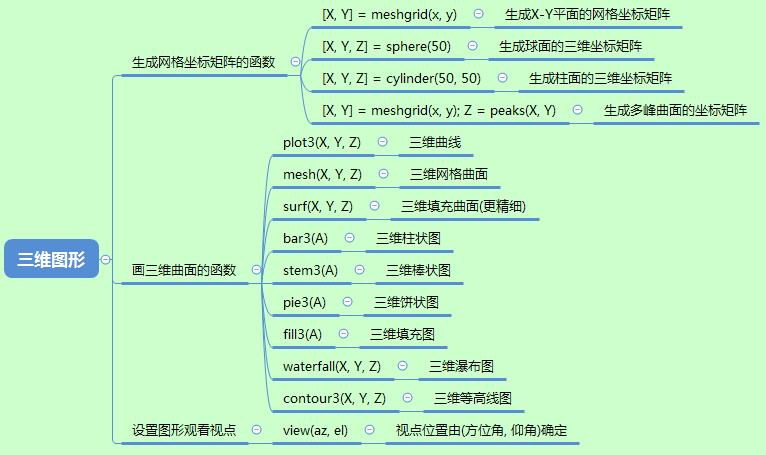代码实现： 【注】每个绘图函数都至少有一个使用实例。注意使用时将代码前面的注释符号%去掉。
clear all; clc;
%---------------------------------------
% t = 0 : pi /100 : 2 * pi;
% x = 8 * cos(t);
% y = 4 * sqrt(2) * sin(t);
% z = -4 * sqrt(2) * sin(t);
% plot3(x, y, z);
% text(0, 0, 0, 'Origin');
% grid on;

%---------------------------------------
% x = 0 : 0.1 : 2 * pi;
% y = 0 : 0.1 : 2 * pi;
% [X, Y] = meshgrid(x, y); % 生成X-Y平面网格坐标矩阵
% Z = cos(X) .* sin(Y); % 生成高度矩阵
% figure(1); plot3(X, Y, Z); %画三维网格曲面
% title('plot3'); xlabel('x'); ylabel('y'); zlabel('z');
%
% figure(2); mesh(X, Y, Z); %画三维网格曲面
% title('mesh'); xlabel('x'); ylabel('y'); zlabel('z');
%
% figure(3); surf(X, Y, Z); %画三维网格曲面
% title('surf'); xlabel('x'); ylabel('y'); zlabel('z');

%-----------------------------------------------------
% [X, Y, Z] = sphere(50);
% subplot(1, 4, 1); surf(X, Y, Z);
%
% [X, Y, Z] = cylinder(50, 50);
% subplot(1, 4, 2); surf(X, Y, Z);
%
% t = 0 : pi / 100 : 2 * pi;
% [X, Y, Z] = cylinder(2 + sin(t), 50);
% subplot(1, 4, 3); surf(X, Y, Z);
%
% [X, Y] = meshgrid(-5 : 0.1 : 5);
% Z = peaks(X, Y);
% subplot(1, 4, 4); surf(X, Y, Z);

%----------------------------------------
% A = [1, 2, 3; 4, 6, 7];
% subplot(2, 2, 1); bar3(A);
% xlabel('x'); ylabel('y'); zlabel('z');
%
% subplot(2, 2, 2); stem3(A);
% xlabel('x'); ylabel('y'); zlabel('z');
%
% A = [0.1, 0.2, 0.3, 0.25, 0.15];
% subplot(2, 2, 3); pie3(A);
%
% subplot(2, 2, 4); fill3(rand(3, 5), rand(3, 5), rand(3, 5), 'b');

%--------------------------------------------------------------------
% [X, Y, Z] = peaks(30);
% subplot(1, 3, 1); waterfall(X, Y, Z);
% title('waterfall'); xlabel('x'); ylabel('y'); zlabel('z');
%
% subplot(1, 3, 2); contour(X, Y, Z, 12); %12为高度等级数
% title('2D-contour'); xlabel('x'); ylabel('y');
%
% subplot(1, 3, 3); contour3(X, Y, Z, 12);
% title('3D-contour'); xlabel('x'); ylabel('y'); zlabel('z');

%-----------------------------------------------------------
[X, Y, Z] = peaks(30);
subplot(1, 3, 1); waterfall(X, Y, Z);
view(-37.5, 30); %设置图形观看视点(方位角, 仰角)
title('az = -37.5, el = 30'); xlabel('x'); ylabel('y'); zlabel('z');

subplot(1, 3, 2); waterfall(X, Y, Z);
view(0, 30);
title('az = 0, el = 30'); xlabel('x'); ylabel('y'); zlabel('z');

subplot(1, 3, 3); waterfall(X, Y, Z);
view(30, 30);
title('az = 30, el = 30'); xlabel('x'); ylabel('y'); zlabel('z');


展开全文MATLAB 三维图形
• 选项 n) 其中每一组 x,y,z 组成一组曲线的坐标参数选项的定义和 plot 函数相同当x,y,z 是同 维向量时则 x,y,z 对应元素构成一条三维曲线当 x,y,z 是同维矩阵时则以 x,y,z 对应列 元素绘制三维曲线曲线条数等于矩阵列...
• 毕业季马上要到了，给大家总结一些我平常用到的奇特但是好用的Matlab画图技巧光滑变化的三维曲面三维曲面展示是目前MATLAB上人眼最容易直观观察的多维矩阵内容一种形式，因此三维矩阵画图在很多的论文里都有涉及。...


毕业季马上要到了，给大家总结一些我平常用到的奇特但是好用的Matlab画图技巧光滑变化的三维曲面
三维曲面展示是目前MATLAB上人眼最容易直观观察的多维矩阵内容一种形式，因此三维矩阵的画图在很多的论文里都有涉及。常用的画图函数有mesh，surf，contourf，plot3等等
因为论文实际打印图片存在固定视角无法旋转的特别，为了详尽的表述一整个二维矩阵的内容，MATLAB中三维绘图除了用Z轴表示数据外，将不同的Z轴对应不同的颜色表示。
下面依次介绍几种三维曲面画图的函数，函数用法建议自行阅读帮助文件。
MESH(X,Y,Z)
Matlab中的曲面绘图都是将XY轴离散化后形状网格，Mesh就是对这个网格再加上Z轴的高度，得到一个彩色一个网状曲面图，如图所示。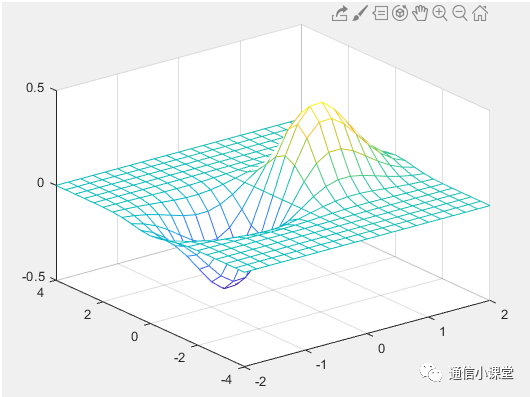通常情况下大家构建的网络比较密，会常用mesh来三维绘图，这样的曲面还是比较好看的，但是如果数据突变比较严重的话这种图会没那么好看，差点意思
SURF(X,Y,Z)
surf是在mesh的基础上给每个网眼的区域填上颜色，然后把线的颜色换成黑色，如图所示。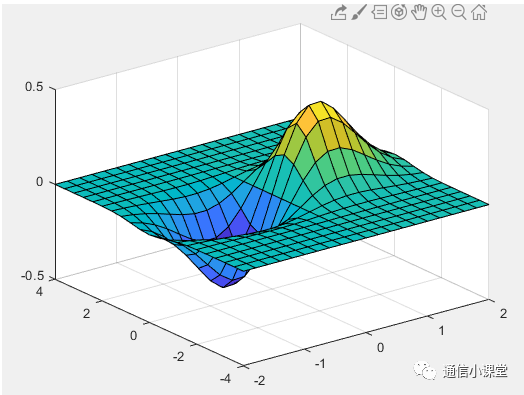如果网格很密黑色曲线也会很密，影响观感，但是去掉黑色曲线，图片也会陷入不利用观察的情况。
Coutour
等高线图，去掉了高度的(类似俯视图)显示改为纯用颜色观察大小，适合进行一些定性的分析。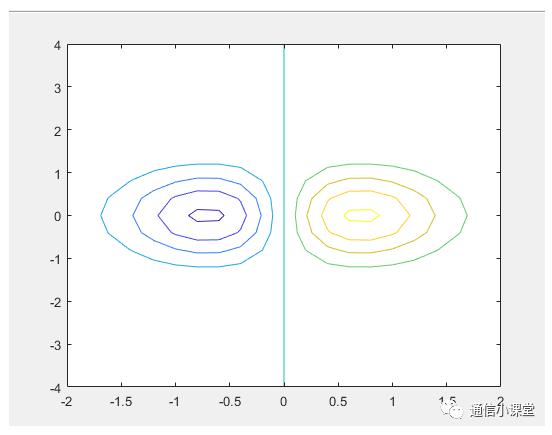上面的图片太空不太适合替代SURF和mesh
CONTOURF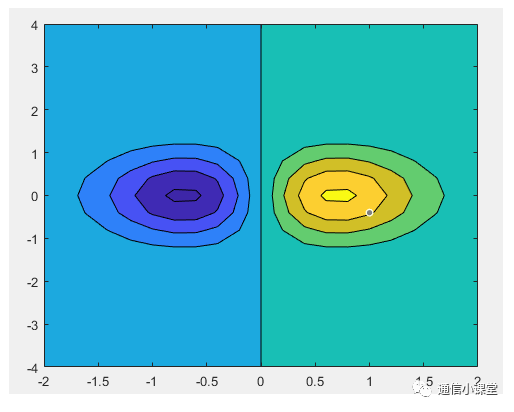为了使得更容易观测，我们对Contourf增加一些配置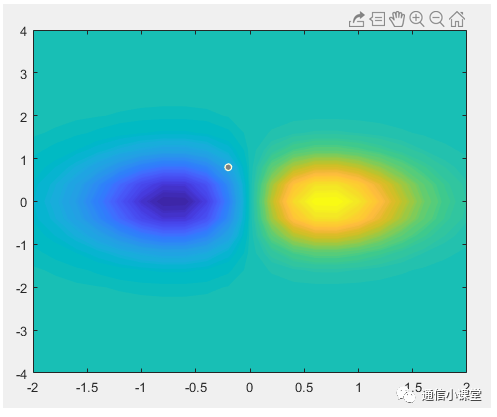就可以得到一副更好看的三维图的显示，

画图代码
[X,Y] = meshgrid(-2:.2:2, -4:.4:4);Z = X .* exp(-X.^2 – Y.^2);figure(1);contourf(X,Y,Z,100,’linestyle’,’none’)


展开全文• ## matlab三维画图总结

万次阅读 2016-11-24 16:50:43
1.画函数的三维图，如：Z(X,Y)=2*X.*exp(-X.^2-Y.^2)+1; close all; [X,Y]=meshgrid(-2:0.5:2,-2:0.5:2);%生成坐标轴 Z=2*X.*exp(-X.^2-Y.^2)+1;%Z是X,Y的函数 %%%%%%%%%%%%%%%%%%%%%%%%%%%%%%%%%%%%%%%%%%%%%%%%%%...
• 基本绘图函数 1、plot(y) 如果y是向量，则采用向量的索引值作为横坐标值，以向量元素的值作为纵坐标值。 如果y是实数矩阵，则相当于对y的每一列进行二...当x为矢量，y是有一与x同维的矩阵时，则以x为共同的横...
• 1 准备好二维矩阵，比如我这有个3x16的矩阵叫SFW1 2 画三维图，一句代码就OK mesh(SFW1) 3 结果如下mesh
• ## matlab三维画图总结

千次阅读 多人点赞 2018-07-13 00:09:42
1、MATLAB中，三维曲线的绘制函数是plot3。语法形式如下： plot3(X, Y, Z） plot3(X, Y, Z, S) plot3(X1, Y1, Z1, S, X2, Y2, Z2, S …)X、Y和Z：三个方向上的坐标值，尺寸相同S：对曲线的线型、颜色、线宽、点...
• 1 目的 将4组三维数据，每组数据画散点图（fig1），拟合出一个曲面（fig2），并将特定Z值的等高线投影到XY平面（fig3）。2 主要函数scatter3( ) 三维散点图scatter3(X,Y,Z,S,C) % 向量 X、Y 和 Z 指定点的位置，都...cell数组
• 最近遇到个问题，如何利用一大堆三维坐标点，拟合出三维曲面。不知道大家有没有遇见过，就是下面这种。我当然是上网百度啊之类的，想看看要怎么作，但转了一圈下来，发现网上几乎是关于下面情况的作图说明，即：我...
• clear;clc close all; load seamount;% 求data的最大最小值 minX = min(x); maxX = max(x);...% 画出一平面 [x1 , y1] = meshgrid(minX:0.01:maxX, minY:0.01:maxY);% 散乱点插值 z1 = griddata(x, y, z,
• 三维画图，大规模的画球,用三维矩阵做，不同颜色表示各种不同的球，里面有word文档详细说明
• ## 使用Matlab绘制三维图的几种方法

万次阅读 多人点赞 2015-08-18 22:27:00
基于Matlab的六个用于绘制三维图像的函数......
• MATLAB绘制三维图形 1.基本的绘图命令 1）plot3函数plot3(x,y,z)%x,y,z为向量或者矩阵 注意x,y,z全为向量时向量长度必须相同 plot3(x,y,z,'b.','MarkerSize',0.5) % MarkerSize 表示点的大小，b.表示绿色的点。 eg...plot3 mesh surf
• round函数 四舍五入，在绘制三维图形时如果数据比较大，可以通过以下方法把数据按应有比例减小 （我自己理解的，有错请指正） x=round(x'./100+1); %这部分使数据减小，便于作图处理等 y=round(...
• 我现在有一个.dat文件，他是一个三维矩阵，如何读取该三维矩阵dat文件
• - 调用画图函数 - heatExplict：显式实现温度矩阵求解函数 ----实现方案均来自于教材。简单说明一下matlab实现。 - Dirichlet boundary - 圆形边界分8种情况讨论，两个临近网格点在圆内的情况有4种，只有一个...
• 2019年4月24日 关于如何把一个三维矩阵里的数据中的一页画成三维图并加密网格。
• ## surf三维画图[matlab]

万次阅读 2017-10-21 10:27:43
surf 三维阴影曲面画图，常用的函数格式 surf(X,Y,Z) X,Y,Z都是矩阵值，规模相等 surf(x,y,Z)x,y是n和m长度的向量，Z是m*n的矩阵(这个也很少用到，最多还是上面那些)代码1:x = -8:0.05:8; y = -3:0.01:5; [X, Y] =...函数 surf
• 强大的绘图功能是Matlab的特点之一，Matlab提供了一系列的绘图函数，用户不需要过多的考虑绘图的细节，只需要给出一些基本参数就能得到所需图形，这类函数称为高层绘图函数。此外，Matlab还提供了直接对图形句柄进行...隐函数作图
• 可以把三维数组的第三维度看成一本书的页，Matlab要显示三维数组就可以将每一页的行和列显示出来，即a(:,:,1)=? a(:,:,2)=? a(:,:,3)=?这就给创建三维数组提供了思路，可以这样定义：b(:,:,1)=[1 2 3;4 5 6]; b(:,:,...
• 使用 matlab 绘制三维图形的方法 三维曲线 plot3 函数与 plot 函数用法十分相似其调用格式为 plot3(x1,y1,z1,选项 1,x2,y2,z2, 选项 2,xn,yn,zn,选项 n)其中每一组 x,y,z 组成一组曲线的坐标参数选项的定义和 plot ...
• ## matlab三维数据绘图

千次阅读 2014-07-15 20:31:45
• 三维画图软件经常会出现下面错误 以mesh(x,y,z)为例： 主要原因是因为没有注意Z数据格式，Z必须是矩阵形式。而且Z矩阵的m*n必须与y,x相关， mesh(X,Y,Z)使用Z确定的颜色绘制线框网格，因此其颜色与曲面高度成...数据维度不一致 mesh
• 写论文，从二维变三维空间，对三维工作空间分析，希望创建漂亮的工作空间示意图。二维区域着色已经在之前博文提到过了：Matlab不规则区域渐变色填充及注意事项。这里主要讲三维曲面着色。 方法 实现绘制曲面的函数有...surf函数使用
• ## mesh三维画图[matlab]

万次阅读 2017-10-21 10:10:32
点这里，可以看surf函数画三维图 mesh是用于画三维的网格图 主要使用格式有： mesh(X,Y,Z) 参数X,Y,Z都是矩阵值规模都是相同的(至于怎么扩展成相同的，一般用的是meshgrid函数设置矩形网络) mesh(x,y,Z)参数x和y...函数 图形 算法
• ## matlab矩阵绘图

万次阅读 2018-01-04 19:13:38
matlab将一个矩阵绘画成三维图像： mesh(m2(1:10000,1:10000))； 其中m2是一个矩阵，之后括号是要显示的矩阵的范围，有时候我们并不需要显示所有的矩阵。 图像保存： saveas(mesh(m1(1:10000,1:10000)),’m1.jpg...
• ## [Matlab科学绘图] Matlab三维绘图总结

千次阅读 多人点赞 2019-06-16 10:25:41
主要对matlab三维绘图函数进行总结并展示一下绘图结果，重点不在函数解读，在于函数介绍，函数的具体使用可以查看matlab的help文档。 函数名 说明 line，plot3，ezplot3 绘制三维曲线 mesh，ezmesh ......

# matlab三维矩阵画图matlab 订阅